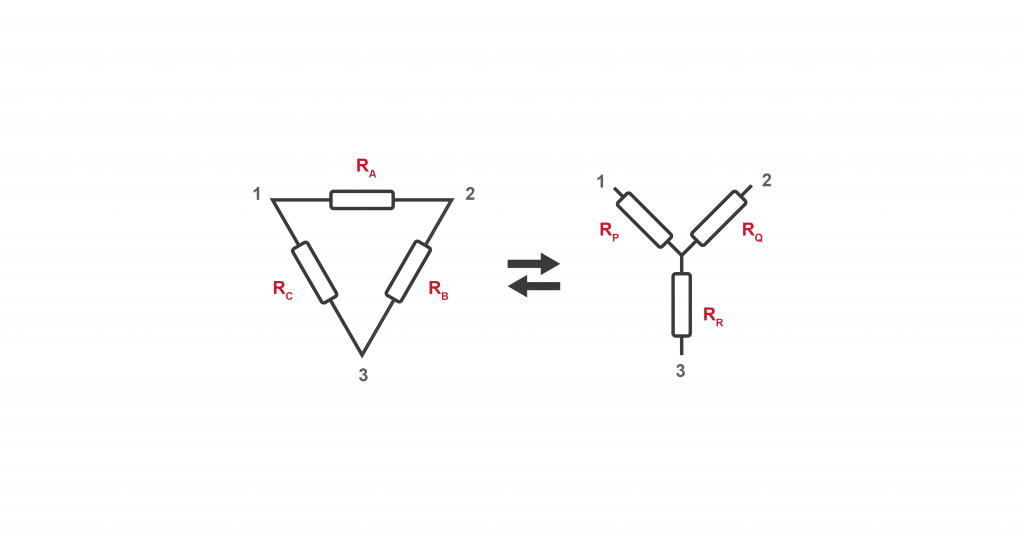Modified: January 12, 2021

# Star Delta Transformation Simplified Method

Start Delta Transformation theorem states that we can convert three-phase configurations Delta to Star and Start to Delta. Here are the simplified equations of the theorem.

There is no need to memorize these equations, there is a little trick behind the generation of these.

## The Trick Behind Delta to Star Transformation

If the resistance of the P is the desired resistor value you need to know, look for the point related to it. In this example, it’s 1. And then go to delta configuration and find the two resistors connected to point 1, and those are resistor A and C. The multiplication of these two resistor values is the denominator

The numerator is the addition of all the three resistors in the delta configuration.

## The Trick Behind Star to Delta Transformation

If you want to find the resistance of A, consider points 1 and 2. If you look at the image below, the desired resistor and the two points are colored in red. And then go to star connection and look for points 1 and 2.  Resistor P and Q are located between these points, so you need to select the opposite resistor, which is the resistance of R. The value of that opposite resistor is the denominator.

The numerator is the same for all three equations and it also has a pattern. It’s the possible ways that you can organize three resistors as pairs. Since the resistors are P, Q, R, we can organize them as PQ, QR, RA.#### Shakila Rathnayake

Shakila Rathnayake is a technology enthusiast, an engineering student, and an editor at MechCollege. Find him on Twitter here. @ShakilaPP